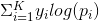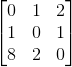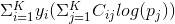Custom categorical cross entropy

#1

I want to define custom cross entropy loss penalizing different class errors.

Categorical cross entropy loss =I want to give different weights to different prediction errors. e.g. For a 3-class problem, below cost matrix is defined.Is it fair to define new cost entropy loss function as below?Where C_{ij} - Cost of classifying class ‘i’ as class ‘j’

0 Likes